## 【AI实战】快速掌握TensorFlow（四）：损失函数 原荐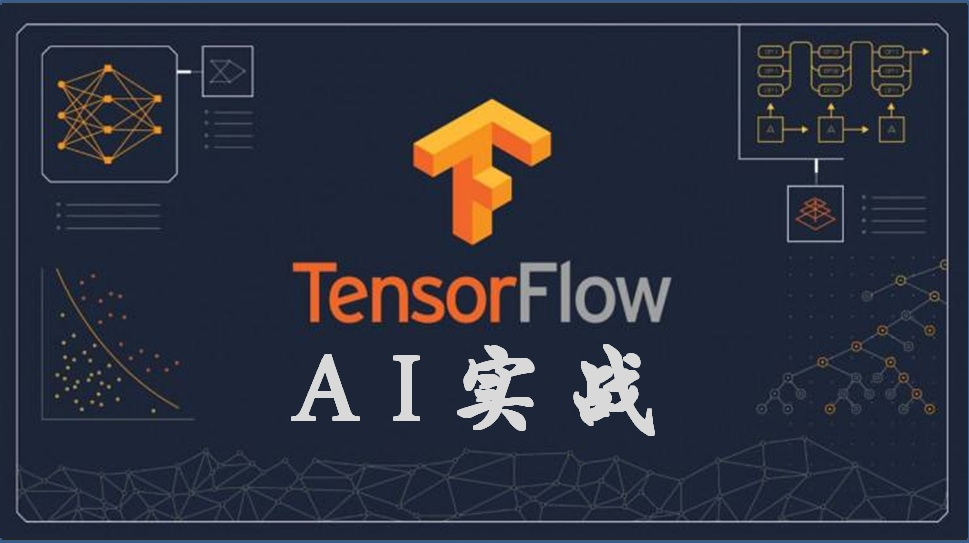1、回归模型的损失函数

``````import tensorflow as tf

sess=tf.Session()

y_pred=tf.linspace(-1., 1., 100)

y_target=tf.constant(0.)``````

（1）L1正则损失函数（即绝对值损失函数）

L1正则损失函数是对预测值与目标值的差值求绝对值，公式如下：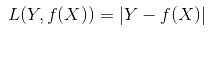``````loss_l1_vals=tf.abs(y_pred-y_target)

loss_l1_out=sess.run(loss_l1_vals)``````

L1正则损失函数在目标值附近不平滑，会导致模型不能很好地收敛。

（2）L2正则损失函数（即欧拉损失函数）

L2正则损失函数是预测值与目标值差值的平方和，公式如下：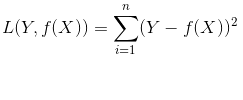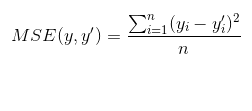``````# L2损失

loss_l2_vals=tf.square(y_pred - y_target)

loss_l2_out=sess.run(loss_l2_vals)

# 均方误差

loss_mse_vals= tf.reduce.mean(tf.square(y_pred - y_target))

loss_mse_out = sess.run(loss_mse_vals)``````

L2正则损失函数在目标值附近有很好的曲度，离目标越近收敛越慢，是非常有用的损失函数。

L1、L2正则损失函数如下图所示：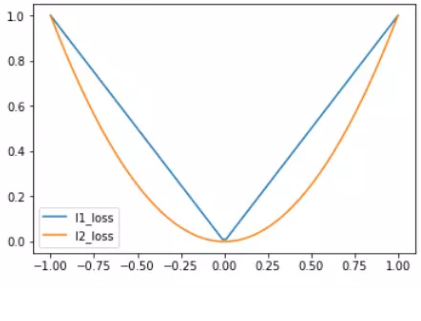（3）Pseudo-Huber 损失函数

Huber损失函数经常用于回归问题，它是分段函数，公式如下：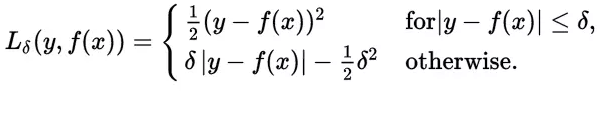Peseudo-Huber损失函数是Huber损失函数的连续、平滑估计，在目标附近连续，公式如下：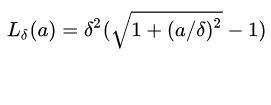``````delta=tf.constant(0.25)

loss_huber_vals = tf.mul(tf.square(delta), tf.sqrt(1. + tf.square(y_target – y_pred)/delta)) – 1.)

loss_huber_out = sess.run(loss_huber_vals)``````

L1、L2、Huber损失函数的对比图如下，其中Huber的delta取0.25、5两个值：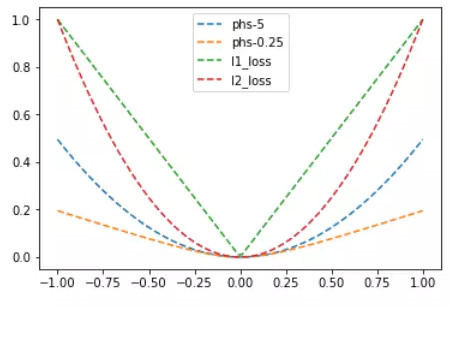2、分类模型的损失函数

``````y_pred=tf.linspace(-3., 5., 100)

y_target=tf.constant(1.)

y_targets=tf.fill([100, ], 1.)``````

（1）Hinge损失函数

Hinge损失常用于二分类问题，主要用来评估向量机算法，但有时也用来评估神经网络算法，公式如下：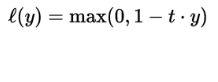``````loss_hinge_vals = tf.maximum(0., 1. – tf.mul(y_target, y_pred))

loss_hinge_out = sess.run(loss_hinge_vals)``````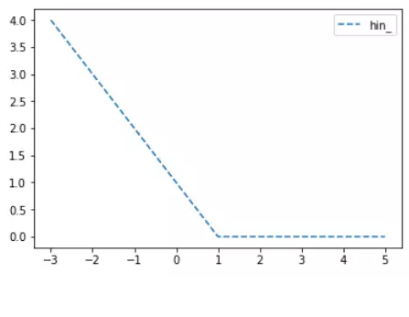（2）两类交叉熵（Cross-entropy）损失函数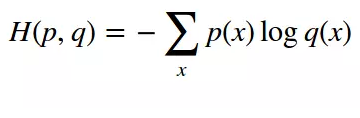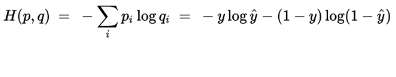``````loss_ce_vals = tf.mul(y_target, tf.log(y_pred)) – tf.mul((1. – y_target), tf.log(1. – y_pred))

loss_ce_out = sess.run(loss_ce_vals)``````

Cross-entropy损失函数主要应用在二分类问题上，预测值为概率值，取值范围为[0,1]，损失函数图如下：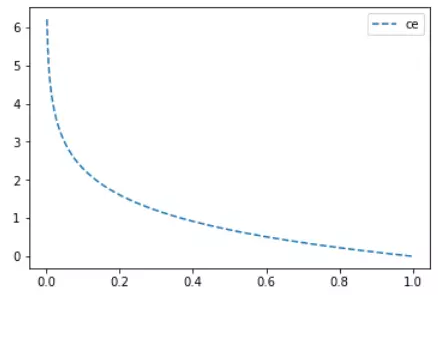（3）Sigmoid交叉熵损失函数

``````loss_sce_vals=tf.nn.sigmoid_cross_entropy_with_logits(y_pred, y_targets)

loss_sce_out=sess.run(loss_sce_vals)``````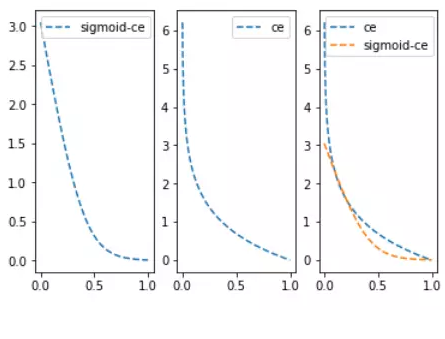（4）加权交叉熵损失函数

``````weight = tf.constant(0.5)

loss_wce_vals = tf.nn.weighted_cross_entropy_with_logits(y)vals, y_targets, weight)

loss_wce_out = sess.run(loss_wce_vals)``````

（5）Softmax交叉熵损失函数

Softmax交叉熵损失函数是作用于非归一化的输出结果，只针对单个目标分类计算损失。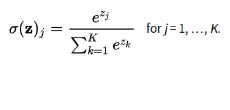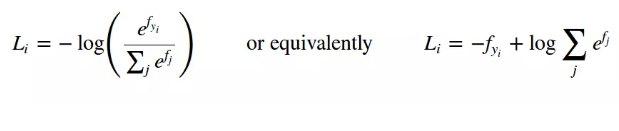``````y_pred=tf.constant([[1., -3., 10.]]

y_target=tf.constant([[0.1, 0.02, 0.88]])

loss_sce_vals=tf.nn.softmax_cross_entropy_with_logits(y_pred, y_target)

loss_sce_out=sess.run(loss_sce_vals)``````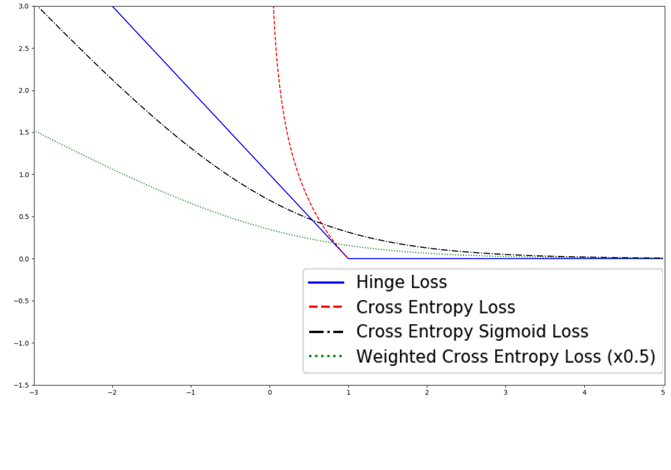3、总结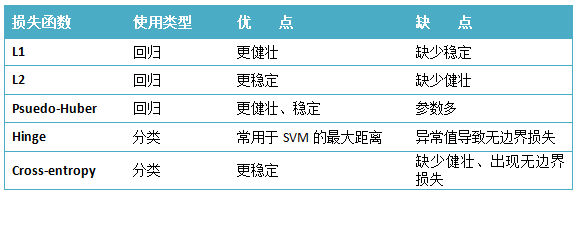© 著作权归作者所有### 雪饼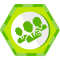【AI实战】快速掌握TensorFlow（三）：激励函数

2018/08/30
1K
0
【AI实战】快速掌握TensorFlow（二）：计算图、会话

2018/08/20
1K
1
【AI实战】快速掌握Tensorflow（一）：基本操作

Tensorflow是Google开源的深度学习框架，来自于Google Brain研究项目，在Google第一代分布式机器学习框架DistBelief的基础上发展起来。Tensorflow于2015年11月在GitHub上开源，在2016年4月补...

2018/08/18
2.3K
0
【AI实战】训练第一个AI模型：MNIST手写数字识别模型

2018/08/11
3.7K
0
【AI实战】手把手教你训练自己的目标检测模型（SSD篇）

2018/08/14
11.1K
25

FAT_mt

4
0
mysql概览

10
0
golang微服务框架go-micro 入门笔记2.2 micro工具之微应用利器micro web

micro web micro 功能非常强大,本文将详细阐述micro web 命令行的功能 阅读本文前你可能需要进行如下知识储备 golang分布式微服务框架go-micro 入门笔记1:搭建go-micro环境, golang微服务框架...

6
0

9
0
Flutter 系列之Dart语言概述

Dart语言与其他语言究竟有什么不同呢？在已有的编程语言经验的基础上，我们该如何快速上手呢？本篇文章从编程语言中最重要的组成部分，也就是基础语法与类型变量出发，一起来学习Dart吧 一、...

6
0